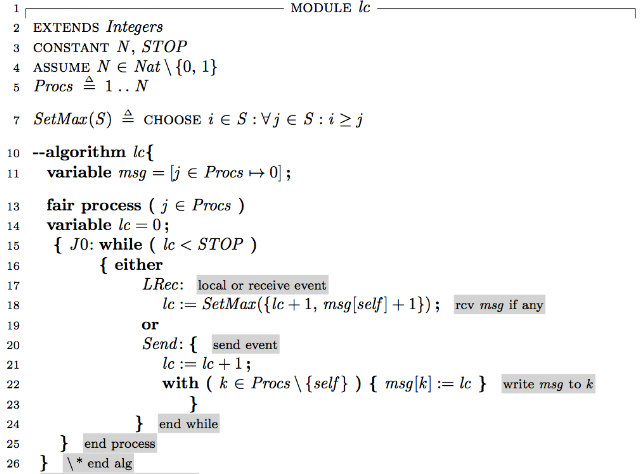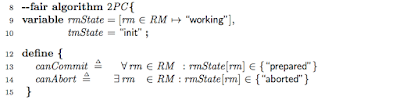## Thursday, January 4, 2018

### Logical clocks and Vector clocks modeling in TLA+/PlusCal

In a distributed system, there is no shared state, counter, or any other kind of global clock.  So we can not implicitly associate an event with its time, because one node may have a different clock than another. Time synchronization is not easy to achieve, and failures complicate things.

It turns out we care about time because of its utility in ordering of the events. Using this observation, in 1978, Leslie Lamport offered a time-free definition for "happens before": Event A happens before event B (denoted as A hb B) if and only if A can causally affect B.

In the context of distributed systems, A hb B iff
1. A and B are on the same node and A is earlier in computation than B
2. A is the send of a message and B is the receive event for that message
3. There is some third event C, which A hb C, and C hb B.

This also suggest the definition for "concurrent" relation. Events A and B are concurrent iff $\neg( A ~hb~ B) \land \neg( B ~hb~ A)$

To capture the hb relation, logical clocks and vector clocks are proposed. I modeled these in PlusCal, and I wanted to share that in this post.

## Logical clocks

A logical clock is a way of assigning number to an event. Assume each node $j$ has a logical clock, $lc.j$, that assigns a number to any event in that node. $lc.j$ can simply be a counter.

We want the following property from $lc$: $A ~hb~ B \Rightarrow lc.A < lc.B$
Note that the converse might not hold.

The algorithm is simple. For a send event at $j$: The clock is incremented and this updated value is the timestamp of the new event and of the message being sent.

For a receive event at $j$: The clock is updated by taking the maximum of the current clock at $j$ and the timestamp of the message (time of the corresponding send event). This maximum must be incremented to ensure that the new clock value is strictly larger than both previous events.

Here is the PlusCal modeling of the algorithm.I use STOP to limit the scope of model checking, often the interesting behavior would present itself in a limited time, we don't need to go to logical clock values of 100s. Another way to do this would be to  go to the model checker advanced options tab, and use depth-first execution, and limit depth.

I don't have interesting invariants to check for logical clocks, because I don't know of a good way to create maintain events with timestamps and also capture the events "causality relationships" and crosscheck that with the event timestamps. Maybe if I had created a time graph, and run the LC model over the time graph that might work.

Instead, I use a bait invariant to get a trace that violates the invariant, so I can observe that the model indeed operates as I intended it to. BaitInv == (\A k \in Procs: lc[k] < STOP /\ msg[k]<STOP) \* violated!

## Vector clocks

With logical clocks, we have $A ~hb~ B \Rightarrow lc.A < lc.B$, and we can not determine whether $A ~hb~ B$ simply by examining their timestamps. For example, for $lc.A_j=3$ and $lc.B_k=9$, what can we say about the relationship between these two events?  We know that $\neg (B ~hb~ A)$ (by the contrapositive of the property above). But do we know that $A ~hb~ B$?

The answer is no. A and B may be events on different processes and B's timestamp might have reached to 9 without any message-chain (causality link) from A. Or not. The process B occurs might indeed have received a message from A or following event, and that might have drove up the lc at that process. The problem with logical clocks is these chains are not captured, because there is only one scalar to keep the counter/clock.

In order to keep a tab on which values were last learned from which processes, we need to extend the scalar counter to be a vector of size N, the number of processes.

The algorithm is then similar to logical clocks algorithm, except for maintaining a vector of "logical clocks".

Send event at $j$: The clock entry of $j$ in the vector clock at $j$ is incremented and the resulting vector is assigned as the timestamp of the event and message being sent.

Receive event at $j$: The vector clock is updated by taking the pair-wise maximum of the current vector and the timestamp of the received message. Then clock entry for $j$ in the vector clock must be incremented to ensure that the new clock value is strictly larger than both previous events.

Here is the PlusCal model of vector clocks algorithm.The PairMax function is neat for defining the pairwise maximum of two vectors of size N. Otherwise, you will notice the model is similar to that of LC.

Again, I am unable to check with respect to events. But here I use this invariant to check that the VC structural property holds. It says, at any point in execution process $k$'s knowledge of its own clock vc[k][k] is greater than or equal to process $l$'s knowledge of $k$'s clock, vc[l][k]. This is because $l$ can only learn of $k$'s clock from a communication chain originating at $k$ and cannot advance $k$'s clock itself.
VCOK == (\A k,l \in Procs: vc[k][k] >= vc[l][k])

(This section is here due to my new year's resolution.)

Of course there is also matrix clocks. While vector clocks maintain what a process knows of the clocks of other processes, matrix clocks maintain what a process knows of what other processes know of other processes as well. "A matrix clock maintains a vector of the vector clocks for each communicating host. Every time a message is exchanged, the sending host sends not only what it knows about the global state of time, but also the state of time that it received from other hosts. This allows establishing a lower bound on what other hosts know, and is useful in applications such as checkpointing and garbage collection." (Frankly I am not aware of matrix clocks being used in practice.)

There is also version vectors, an application of the vector clocks idea to distributed replica maintenance: instead of having N to be the number of clients/processes, N becomes the number of replicas, which is a smaller number, 3-5. Version vectors enable causality tracking among data replicas and are a basic mechanism for optimistic replication.

I wonder if we could have version matrices, that extends version vectors  analogous to how matrix clocks extend vector clocks. Would that have any utility for distributed replicas?

Earlier I had provided a PlusCal model of hybrid logical clocks that augment logical clocks with physical clocks.

Here is some previous discussion/context about why I started assigning TLA+/PlusCal modeling projects in distributed systems classes.

Clicking on label "tla" at the end of the post you can reach all my posts about TLA+

#### 1 comment:

eu4 console commands said...

this discussion is very good very academic and theoretically coherent and holistic explanation. yet the sound quality is not that good but i can't complain tho. thank you

### Two-phase commit and beyond

In this post, we model and explore the two-phase commit protocol using TLA+. The two-phase commit protocol is practical and is used in man...